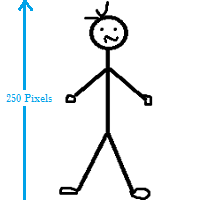# Uncertainty in Statistics

Share onThis stick man is about 250 pixels tall. A tiny amount of error (perhaps one half of a pixel) is built in.

In the real word, uncertainty (sometimes called error or bias) is a part of everyday life, but in statistics we try to quantify just how much uncertainty is in our experiment, survey or test results.

The two main types are epistemic (things we don’t known because of a lack of data or experience) and aleatoric (things that are simply unknown, like what number a die will show on the next roll).

It is measured through a variety of ways.

## Measures of Uncertainty

The confidence interval (CI) shows what the uncertainty is with a certain statistic (e.g. a mean). The margin of error is a range of values above and below a confidence interval’s sample statistic. For example, a survey might report a 95% confidence level of between 4.88 and 5.26. That means if the survey is repeated using the same methods, 95% of the time the true population parameter will fall between 4.88 and 5.26, 95% of the time.

The mean error refers to the mean (average) of all errors. “Error” in this context is the difference between a measured and true value.

For what happens to measurement errors when you use uncertain measurements to calculate something else (For example, using length to calculate area), see: Propagation of Uncertainty. In general terms, relative precision shows uncertainty as a fraction of a quantity. It is the ratio of a measurement’s precision and the measurement itself.

For the entropy of a distribution where a row variable X explains a column variable Y, see: Uncertainty Coefficient.

## Sources of Uncertainty

• Interpolation errors happen because of a lack of data, and may be compounded by your choice of interpolation method.
• Model bias happens because any model is an approximation, or a best guess at what a true distribution might look like.
• Numerical errors are human errors that creep in when translating mathematical models into a computer.
• Observational error is due to the variability of measurements in an experiment.
• Parameter uncertainty happens because we don’t know the exact, or “best” values in a population—we can only take a good guess with sampling.

## References

Everitt, B. S.; Skrondal, A. (2010), The Cambridge Dictionary of Statistics, Cambridge University Press.
Levine, D. (2014). Even You Can Learn Statistics and Analytics: An Easy to Understand Guide to Statistics and Analytics 3rd Edition. Pearson FT Press
Potter, K. (2012). Uncertainty and Parameter Space Analysis in Visualization. Scientific Computing and Imaging Institute University of Utah. Retrieved April 9, 2019 from: http://www.sci.utah.edu/~kpotter/talks/StatisticalUncertainty.pdf

CITE THIS AS:
Stephanie Glen. "Uncertainty in Statistics" From StatisticsHowTo.com: Elementary Statistics for the rest of us! https://www.statisticshowto.com/uncertainty-in-statistics/
---------------------------------------------------------------------------Need help with a homework or test question? With Chegg Study, you can get step-by-step solutions to your questions from an expert in the field. Your first 30 minutes with a Chegg tutor is free!